# which is larger f (0) or g (0).### Precalculus: Mathematics for Calcu...

6th Edition
Stewart + 5 others
Publisher: Cengage Learning
ISBN: 9780840068071### Precalculus: Mathematics for Calcu...

6th Edition
Stewart + 5 others
Publisher: Cengage Learning
ISBN: 9780840068071

#### Solutions

Chapter 2.3, Problem 8E

a.

To determine

## To calculate: which is larger f (0) or g (0).

Expert Solution

f(0) is larger.

### Explanation of Solution

Given information: the graph of function g and f is provided,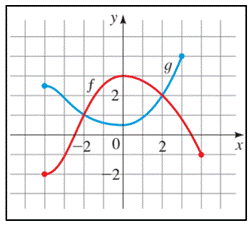Graph: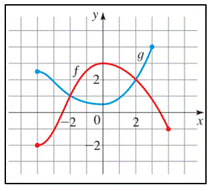In the graph of g and f , the value of g(0) and f(0) is the corresponding y- value at x = 0 , that is,

f(0)=3g(0)=0.5

By comparing both the y -values,

f(0)>g(0)

Hence, f(0) is larger.

b.

To determine

### To calculate: which is larger f ( −3 ) or g (3).

Expert Solution

g(3) is larger.

### Explanation of Solution

Given information: the graph of function g and f is provided,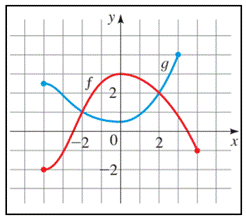Graph: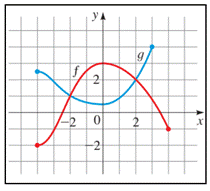In the graph of g and f , the value of g(3) and f(3) is the corresponding y- value at x = 3,3 , that is,

f(3)=1g(3)=2

By comparing both the y -values,

f(3)<g(3)

Hence, g(3) is larger.

c.

To determine

### To calculate: where f(x)=g(x)

Expert Solution

f(x)=g(x) at x=2 and x = 2.

### Explanation of Solution

Given information: the graph of function f and g is provided,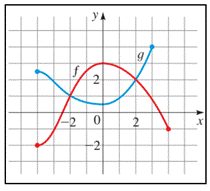Graph:Calculation:

The function values(s) where f(x)=g(x) is the point where both the lines intersect each other,

Therefore, from the graph f(x)=g(x) at,

x=2x=2

hence, f(x)=g(x) at x=2 and x = 2.

### Have a homework question?

Subscribe to bartleby learn! Ask subject matter experts 30 homework questions each month. Plus, you’ll have access to millions of step-by-step textbook answers!